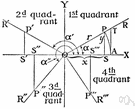trigonometry

(redirected from Pretrigonometry)
Also found in: Thesaurus, Encyclopedia.

trig·o·nom·e·try

(trĭg′ə-nŏm′ĭ-trē)
n.
The branch of mathematics that deals with the relationships between the sides and the angles of triangles and the calculations based on them, particularly the trigonometric functions.

[New Latin trigōnometria : Greek trigōnon, triangle; see trigon + Greek -metriā, -metry.]

trig′o·no·met′ric (-nə-mĕt′rĭk), trig′o·no·met′ri·cal (-rĭ-kəl) adj.

trigonometry

(ˌtrɪɡəˈnɒmɪtrɪ)
n
(Mathematics) the branch of mathematics concerned with the properties of trigonometric functions and their application to the determination of the angles and sides of triangles. Used in surveying, navigation, etc. Abbreviation: trig
[C17: from New Latin trigōnometria from Greek trigōnon triangle]

trig•o•nom•e•try

(ˌtrɪg əˈnɒm ɪ tri)

n.
the branch of mathematics that deals with the relations between the sides and angles of plane or spherical triangles, and the calculations based on them.
[1605–15; < New Latin trigōnometria; see trigon, -o-, -metry]
trig`o•no•met′ric (-nəˈmɛ trɪk) trig`o•no•met′ri•cal, adj.

trig·o·nom·e·try

(trĭg′ə-nŏm′ĭ-trē)
The study of the properties and uses of trigonometric functions.

trigonometry

the branch of mathematics that treats the measurement of and relationships between the sides and angles of plane triangles and the solid figures derived from them. — trigonometric, trigonometrical, adj.
ThesaurusAntonymsRelated WordsSynonymsLegend:
 Noun 1trigonometry - the mathematics of triangles and trigonometric functionstrigmath, mathematics, maths - a science (or group of related sciences) dealing with the logic of quantity and shape and arrangementpure mathematics - the branches of mathematics that study and develop the principles of mathematics for their own sake rather than for their immediate usefulnessspherical trigonometry - (mathematics) the trigonometry of spherical trianglestriangulation - a trigonometric method of determining the position of a fixed point from the angles to it from two fixed points a known distance apart; useful in navigation
Translations
trigonometrie
trigonometria
trigonometri

trigonometry

[ˌtrɪgəˈnɒmɪtrɪ] N

trigonometry

[ˌtrɪgəˈnɒmətri] n

trigonometry

[ˌtrɪgəˈnɒmɪtrɪ] ntrigonometria
Site: Follow: Share:
Open / Close